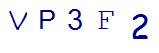### 4th Grade Math Help: 5 Ways to Conquer Factors and Multiples

After kids learn multiplication, a typical 4th grade math curriculum then dives into factors and multiples....

### How to Give Your Child Geometry Help, Teach Proofs like a Math Tutor

Geometry is the branch of mathematics concerned with the properties and relations of points, lines, surface...

### 5 Quick Math Drills to Use with Your 4th Grade Math Student

Fourth grade is a key year for a child’s math education. Granted, every grade in elementary school math is ...

### How to Master 3rd Grade Division Using Free Math Worksheets

Third-grade is an important year in math development as children expand their understanding of multiplicati...